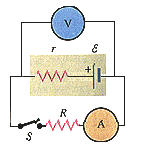# Circuit Problem involving resistors and a switch

yaro99

## Homework Statement

When switch S in the figure below is open, the voltmeter V of the battery reads 3.68 V. When the switch is closed, the voltmeter reading drops to 3.50 V, and the ammeter A reads 1.80 A. Assume that the two meters are ideal, so they don't affect the circuit.

Find the emf of the battery.

Find the internal resistance of the battery.

Find the circuit resistance R.## Homework Equations

V=IR
Resistors in series: Req=R1+R2+R3...

## The Attempt at a Solution

I found the emf of the battery to be 3.68 V, because it is the only voltage source being read by the voltmeter when the switch is open.

I am not sure how to find the resistance.
I have come up with this:
Req = r + R
Vtotal = I*Req ==> Req = Vtotal/I = 3.5/1.8 = 1.944 Ω

1.944 = r + R

I am not sure where to go from here.

## Answers and Replies

Homework Helper
Gold Member
I found the emf of the battery to be 3.68 V, because it is the only voltage source being read by the voltmeter when the switch is open.

Right.
I am not sure how to find the resistance.
I have come up with this:
Req = r + R
Vtotal = I*Req ==> Req = Vtotal/I = 3.5/1.8 = 1.944 Ω

1.944 = r + R

I am not sure where to go from here.

What's the voltage read by the voltmeter after the switch is closed?

yaro99
Right.

What's the voltage read by the voltmeter after the switch is closed?

It will be 3.5 V according to the problem, which I tried using in my calculations. I'm still confused.

Homework Helper
Gold Member
It will be 3.5 V according to the problem, which I tried using in my calculations. I'm still confused.

OK, so how about you write an equation relating that 3.5V with the current of 1.8A, the battery voltage E and the unknown resistor r?

Then, do you have 2 equations with 2 unknowns (r and R)?

Homework Helper
Gold Member
The emf of the battery is 3.68 V. If you could connect the voltmeter to the right of r it would still read that when current is flowing. But connected to the left there is a voltage drop across r. How much is it?

When you know that, since you know the current through r, you can calculate r.

You will also know the voltage drop across R, the current is the same and known so you can calculate R.